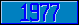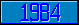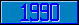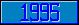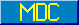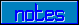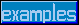## \$%CRCCCITT^STRING

### M[UMPS] by Example

Approved for inclusion in a future M[UMPS] language standard as part of the library for cyclic redundancy checks.

This function computes a Cyclic Redundancy Code of the 8-bit character string string, using X16 + X12 + X5 + 1 as the polynomial. The optional parameter seed may supply an initial value, which allows for running CRC calculations on multiple strings. If the parameter seed is not specified, a default value of 65,535 (216–1) is assumed. The value of seed is limited to 0 ≤ seed < 216. The function value will be between 0 and 216.

A typical method of transmitting data is to send data followed by a check-sum value, e.g.:

```

Set CRC=\$Extract(Data,L–1,L)

Set VAL=0

For i=1:1:2 Set VAL=VAL*256+\$ASCII(CRC,i)

Set DATA=\$Extract(Data,1,L–2)

If \$%CRCCCITT^STRING(Data)'=VAL Do Error

```

A check-sum can also be calculated over multiple strings:

```
Set(I,C)=0

For  Set I=\$Order(X(I)) Quit:'I  Do

. Set C=\$%CRCCCITT^STRING(X(I),C)

```
Reference Value \$%CRCCCITT^STRING(123) 23502 \$%CRCCCITT^STRING(1234) 21321 \$%CRCCCITT^STRING(12345) 17760 \$%CRCCCITT^STRING(" ") 50578 \$%CRCCCITT^STRING("1234567890123456789012345678901234567890") 51307 \$%CRCCCITT^STRING("ABCDEFGHIJKLMNOPQRSTUVWXYZ") 55521 \$%CRCCCITT^STRING("a") 40311 \$%CRCCCITT^STRING("abc") 20810 \$%CRCCCITT^STRING("abcd") 11510 \$%CRCCCITT^STRING("abcdef") 13549 \$%CRCCCITT^STRING("abcdefghijklmnopqrstuvwxyz") 21474

The MDC has approved code to approximate the return value of this function. Implementors are encouraged to provide more accurate and efficient code).